##### Circuit Analysis For Dummies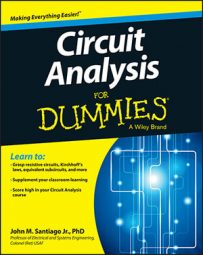There’s a special op amp circuit —a differential amplifier, or subtractor — that is actually a combination of a noninverting amplifier and inverting amplifier. A differential amplifier multiplies the difference between two voltages.

Here is an op amp subtractor.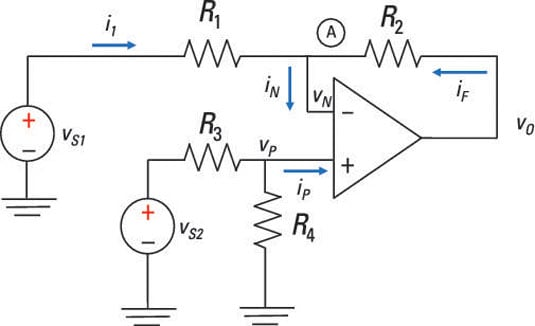You use superposition to determine the input and the output relationship. The superposition technique involves the following steps:

1. Turn on one source and turn off the others.

2. Determine the output of the source that’s on.

3. Repeat for each input, taking the sources one at a time.

4. Algebraically add up all the output contributions for each input to get the total output.

For sample circuit, first turn off voltage source vS2 so that there’s no input at the noninverting terminal (vP = 0). With the noninverting input grounded, the circuit acts like an inverting amplifier. You wind up with output contribution vO1 due to vS1: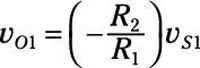You next turn off voltage source vS1 (vN = 0) and turn vS2 back on. The circuit now acts like a noninverting amplifier. Because this is an ideal op amp, no current (iP = 0) is drawn from the series connection of resistors R3 and R4, so you can use the voltage divider equation to determine vP. The voltage at the noninverting input is given by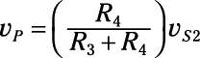The noninverting input vP is amplified to give you an output vO2: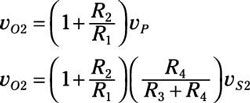You then add up the outputs vO1 and vO2 to get the total output voltage: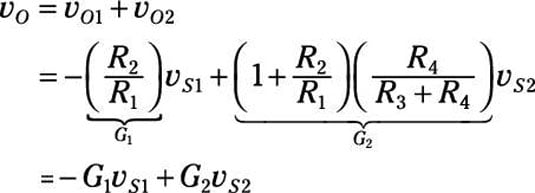Here, –G1 is the inverting gain and G2 is the noninverting gain. You need the following constraint to form a subtractor: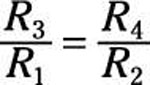Applying the constraint simplifies the output voltage, giving you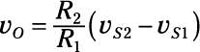There you have it! You now have the output proportional to the difference between the two inputs.